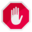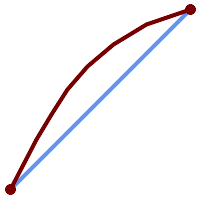## Name

ST_Segmentize — Returns a modified geometry/geography having no segment longer than a given distance.

## Synopsis

`geometry ST_Segmentize(`geometry geom, float max_segment_length`)`;

`geography ST_Segmentize(`geography geog, float max_segment_length`)`;

## Description

Returns a modified geometry/geography having no segment longer than `max_segment_length`. Length is computed in 2D. Segments are always split into equal-length subsegments.

• For geometry, the maximum length is in the units of the spatial reference system.

• For geography, the maximum length is in meters. Distances are computed on the sphere. Added vertices are created along the spherical great-circle arcs defined by segment endpoints.This only shortens long segments. It does not lengthen segments shorter than the maximum length.For inputs containing long segments, specifying a relatively short `max_segment_length` can cause a very large number of vertices to be added. This can happen unintentionally if the argument is specified accidentally as a number of segments, rather than a maximum length.

Availability: 1.2.2

Enhanced: 3.0.0 Segmentize geometry now produces equal-length subsegments

Enhanced: 2.3.0 Segmentize geography now produces equal-length subsegments

Enhanced: 2.1.0 support for geography was introduced.

Changed: 2.1.0 As a result of the introduction of geography support, the usage `ST_Segmentize('LINESTRING(1 2, 3 4)', 0.5)` causes an ambiguous function error. The input needs to be properly typed as a geometry or geography. Use ST_GeomFromText, ST_GeogFromText or a cast to the required type (e.g. `ST_Segmentize('LINESTRING(1 2, 3 4)'::geometry, 0.5) )`

## Examples

Segmentizing a line. Long segments are split evenly, and short segments are not split.

```SELECT ST_AsText(ST_Segmentize(
'MULTILINESTRING((0 0, 0 1, 0 9),(1 10, 1 18))'::geometry,
5 ) );
---------------------------------------------------
MULTILINESTRING((0 0,0 1,0 5,0 9),(1 10,1 14,1 18))
```

Segmentizing a polygon:

```SELECT ST_AsText(
ST_Segmentize(('POLYGON((0 0, 0 8, 30 0, 0 0))'::geometry), 10));
-------------------------------------------------------
POLYGON((0 0,0 8,7.5 6,15 4,22.5 2,30 0,20 0,10 0,0 0))
```

Segmentizing a geographic line, using a maximum segment length of 2000 kilometers. Vertices are added along the great-circle arc connecting the endpoints.

```SELECT ST_AsText(
ST_Segmentize(('LINESTRING (0 0, 60 60)'::geography), 2000000));
-------------------------------------------------------------
LINESTRING(0 0,4.252632294621186 8.43596525986862,8.69579947419404 16.824093489701564,13.550465473227048 25.107950473646188,19.1066053508691 33.21091076089908,25.779290201459894 41.01711439406505,34.188839517966954 48.337222885886,45.238153936612264 54.84733442373889,60 60)
```A geographic line segmentized along a great circle arc